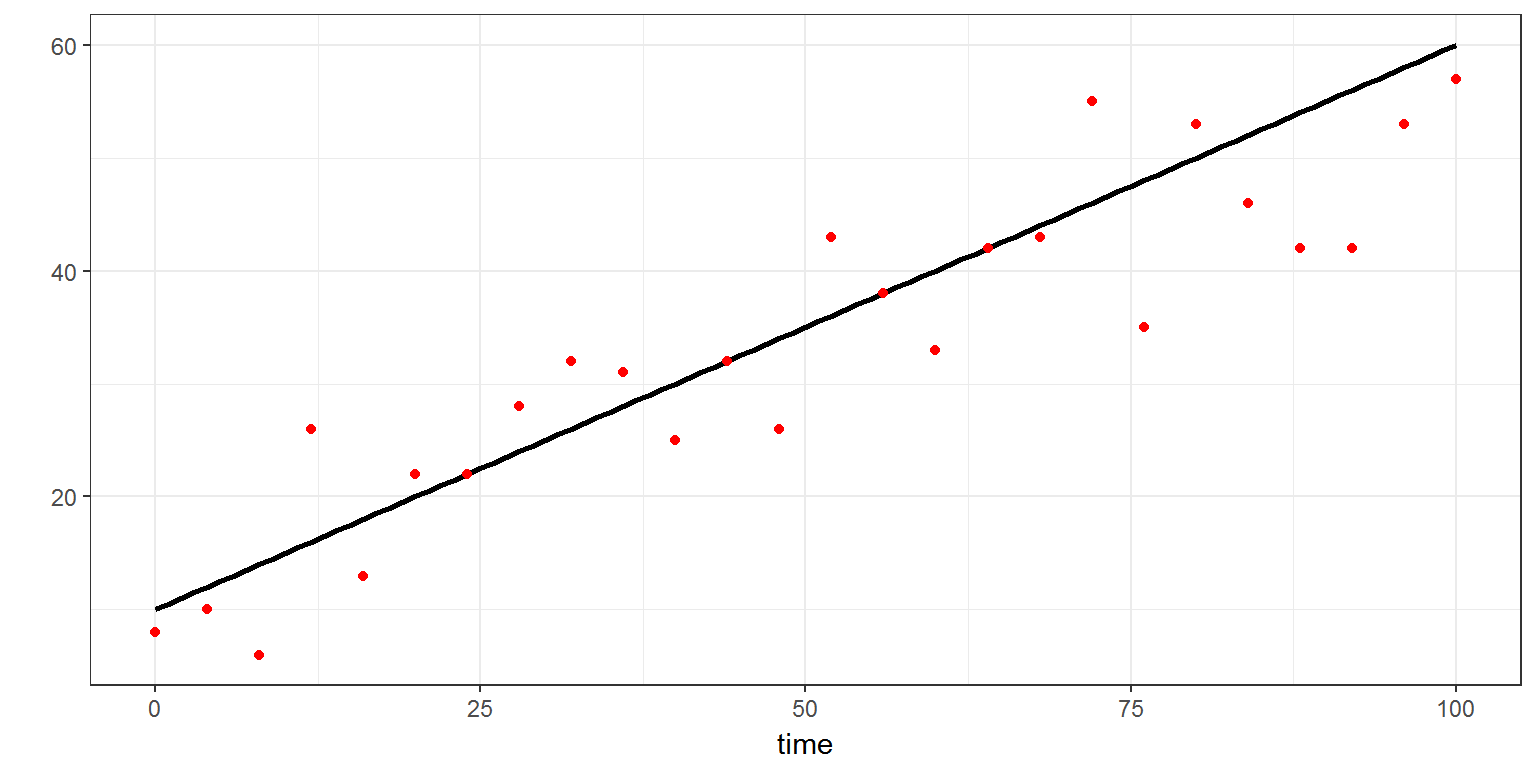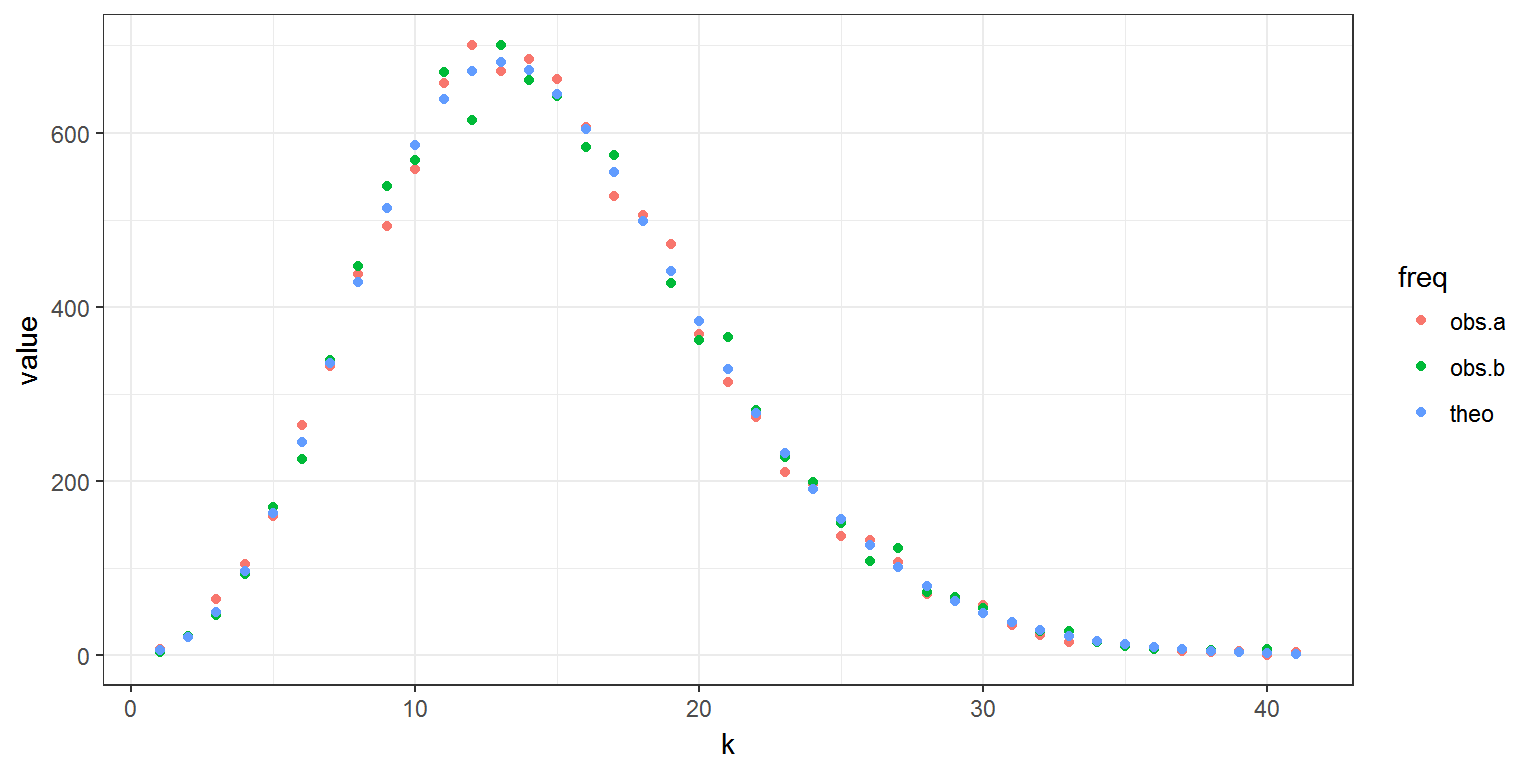R script: count.R

Mlxtran code: model/count.txt

$$\newcommand{\esp}{\mathbb{E}\left(#1\right)} \newcommand{\var}{\mbox{Var}\left(#1\right)} \newcommand{\deriv}{\dot{#1}(t)} \newcommand{\prob}{ \mathbb{P}\!(#1)} \newcommand{\eqdef}{\mathop{=}\limits^{\mathrm{def}}} \newcommand{\by}{\boldsymbol{y}} \newcommand{\bc}{\boldsymbol{c}} \newcommand{\bpsi}{\boldsymbol{\psi}} \def\pmacro{\texttt{p}} \def\like{{\cal L}} \def\llike{{\cal LL}} \def\logit{{\rm logit}} \def\probit{{\rm probit}} \def\one{{\rm 1\!I}} \def\iid{\mathop{\sim}_{\rm i.i.d.}} \def\simh0{\mathop{\sim}_{H_0}} \def\df{\texttt{df}} \def\res{e} \def\xomega{x} \newcommand{\argmin}{{\rm arg}\min_{#1}} \newcommand{\argmax}{{\rm arg}\max_{#1}} \newcommand{\Rset}{\mbox{\mathbb{R}}} \def\param{\theta} \def\setparam{\Theta} \def\xnew{x_{\rm new}} \def\fnew{f_{\rm new}} \def\ynew{y_{\rm new}} \def\nnew{n_{\rm new}} \def\enew{e_{\rm new}} \def\Xnew{X_{\rm new}} \def\hfnew{\widehat{\fnew}} \def\degree{m} \def\nbeta{d} \newcommand{\limite}{\mathop{\longrightarrow}\limits_{#1}} \def\ka{k{a}} \def\ska{k{\scriptscriptstyle a}} \def\kel{k{e}} \def\skel{k{\scriptscriptstyle e}} \def\cl{C{\small l}} \def\Tlag{T\hspace{-0.1em}{lag}} \def\sTlag{T\hspace{-0.07em}{\scriptscriptstyle lag}} \def\Tk{T\hspace{-0.1em}{k0}} \def\sTk{T\hspace{-0.07em}{\scriptscriptstyle k0}} \def\thalf{t{1/2}} \newcommand{\Dphi}{\partial_\pphi #1} \def\asigma{a} \def\pphi{\psi} \newcommand{\stheta}{{\theta^\star}} \newcommand{\htheta}{{\widehat{\theta}}}$$

# 1 Introduction

Longitudinal count data is a special type of longitudinal data that can take only nonnegative integer values $$\{0, 1, 2,\ldots\}$$ that come from counting something, e.g., the number of seizures, hemorrhages or lesions in each given time period.

Considering the observations $$(y_{j},\, 1 \leq j \leq n)$$ as a sequence of conditionally independent random variables, the model is completely defined by the probability mass functions $\prob{y_{j}=k} \quad \text{for} \ k=0, 1, 2,\ldots \ \text{and} \ 1 \leq j \leq n$

The distribution of count data can be defined in the block DEFINITIONof the Section [LONGITUDINAL]using the probability mass functions.

# 2 Examples

## 2.1 Example 1: Poisson distribution

In this example, the Poisson distribution is used for defining the distribution of $$y_{j}$$: $y_j \sim \text{Poisson}(\lambda_j)$ where the Poisson intensity $$\lambda_j$$ is function of time: $\lambda_j = a + b \, t_j$

This model is first implemented using the Mlxtran library of distributions:

poissonModela <- inlineModel("
[LONGITUDINAL]
input =  {a,b}

EQUATION:
lambda = a +b*t

DEFINITION:
y = {distribution=poisson, lambda=lambda}
")

We will use simulx for computing $$\lambda$$ every hour between 0h and 100h and for generating observations $$(y_j)$$ every 2 hours between 0h and 100h, with $$a=10$$ and $$b=0.5$$.

p <- c(a=10, b=0.5)
l <- list(name='lambda', time=seq(0, 100, by=1))
y <- list(name='y', time=seq(0, 100, by=4))

res1a <- simulx(model=poissonModela,
parameter=p,
output=list(l, y))

we can now plot the Poisson intensity and the simulated data:

print(ggplot(aes(x=time, y=lambda), data=res1a$lambda) + geom_line(size=1) + geom_point(aes(x=time, y=y), data=res1a$y, color="red") + ylab("") )Remark: instead of using the library of distributions it is possible to define the probability mass function in the Mlxtran code:

poissonModelb <- inlineModel("
[LONGITUDINAL]
input =  {a,b}

EQUATION:
lambda=a +b*t

DEFINITION:
y = {type=count, P(y=k)=exp(-lambda)*(lambda^k)/factorial(k)}
")

Both implementations are equivalent.

## 2.2 Example 2: Negative binomial distribution

Suppose there is a sequence of independent Bernoulli trials, each trial having two potential outcomes called “success” and “failure”. In each trial the probability of success is $$p$$ and of failure is $$(1-p)$$. We are observing this sequence until a predefined number $$n$$ of failures has occurred. Then the random number of successes, $$y$$, we have seen will have the negative binomial distribution.

For $$k=0,1,2,\ldots$$,

$\prob{y=k} = \left( \begin{array}{c} k+n-1 \\ k \end{array} \right)p^k (1-p)^n$

Let us use simulx for drawing a random sample of size $$N=10000$$ from a negative binomial distribution:

negbinModela <- inlineModel("
[LONGITUDINAL]
input =  {n,p}
DEFINITION:
y = {distribution=negativeBinomial, size=n, prob=p}
")

param <- c(n=10,p=0.4)
N <- 10000
y <- list(name='y', time=seq(1, N, by=1))

res2a <- simulx(model=negbinModela,
parameter=param,
output=y)

We can use equivalently define the probability mass function in the Mlxtran code:

negbinModelb <- inlineModel("
[LONGITUDINAL]
input =  {n,p}
DEFINITION:
y = {type   = count,
P(y=k) = factorial(k+n-1)/factorial(k)/factorial(n-1)*((1-p)^k)*(p^n)
}
")

res2b <- simulx(model=negbinModelb,
parameter=param,
output=y)

We can now compare the empirical distribution of these 2 samples with the theoretical negative binomial distribution:

library(plyr)
library(reshape2)

ca=count(res2a$y$y)
cb=count(res2b$y$y)
names(ca) <- c('k','obs.a')
names(cb) <- c('k','obs.b')
c <- merge(ca, cb)
c$theo <- dnbinom(c$k,size=param,p=param)*N
r <- melt(c,  id = 'k', variable.name = 'freq')
print(ggplot(r, aes(k,value, colour=freq)) + geom_point())